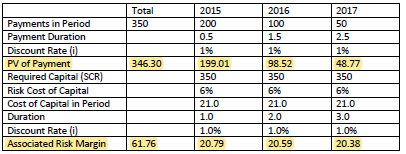# 2015 Fall#19

Does it make sense that the examiner's solution for this problem doesn't take the average of payments like we see in the GAAP Goodwill cost of capital approach? Also, in my first attempt at this problem, I summed all payments for the 2015 liabilities calculation then successively subtracted payments in order to have the whole unpaid amount (350 for 2015, 150 for 2016, 50 for 2017), but that was not correct either. Is there something that I'm missing? Thanks!

## Comments

• Hm. I think you may be mixing up the loss payments and the capital a bit? See if this makes sense or not:

The SCR from the 99.5th percentile is 350 and "held to the end of the period" so that's 350 each year for a cost of capital of 350*6%=21 per year to be discounted from the end of each year.

It's NOT directly related to the liability payment schedule. (The fact that the payments happen to add up to \$350 makes it easy to get mixed up here though!) Each payment is discounted from the middle of its year per the payment assumption, e.g. the first payment of 200 is discounted half a year.

• I'm not sure I'm following. To be more specific, I (maybe mistakenly) thought that for both the goodwill problem (2016 Fall#18) and the Solvency II problem (2015 Fall#19), one of the calculations that is required is the capital required to support liabilities. In the goodwill problem, we have to take the total unpaid amounts and decrease them each year by the paid amounts from the previous year. However, in the the Solvency II problem, it looks like we're using only the payments. Why is this?

In both problems, isn't the goal to calculate an estimate of liabilities? (I realize this is only one part of the Solvency II problem, and we must also calculate the risk margin, but the risk margin calculation is straightforward to me.)

• You're correct that in both cases we need the capital, but the required amount is determined differently.

In 2016F#18, the required capital is defined as 50% of the average nominal unpaid loss + LAE over the previous 12 months.

For 2015F#19, under Solvency II, the required capital is the SCR (derived from the 99.5th percentile of our model).

• Hi @jstark3572,

The comments from @PaulKory are accurate so I hope my comments below don't add to any confusion, but here's another way of thinking about the solution: The table given in the solution is doing 2 separate calculations at the same time and if I had been solving this problem, I probably would have split it into 2 separate tables for clarity.

• The first 4 rows of the table are for calculating the best estimate of the PV of the liabilities.
• The 6 rows below that are for calculating the risk margin.You mentioned that the risk margin calculation makes sense - your question is then about the top 4 rows where PV(liabilities) is calculated. If you isolate this and compare it to Alice's solution for 2016.Fall Q18 you'll see that for PV(liabilities), both are discounting the amount paid in each year. These yearly discounted amounts are then summed across all years to get the total best estimate of PV(liabilities).

Note that the risk margin calculation is a little different between 2016.Fall Q18 and 2015.Fall Q19. So getting back to the very first sentence of your first post, for the goodwill problem, they aren't taking the average of payments here, they're taking the average of 50% of the unpaid amounts. You have to be very clear on the separation between calculating PV(liabilities) and calculating the risk margin because those are 2 different things. (And in the 2015 problem, it didn't help that the SCR value of 350 was coincidentally the same as the total unpaid amounts.)

Overall, the 2015 problem was a challenging problem, as was mentioned in the examiner's report.

Sign In or Register to comment.